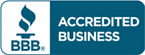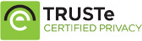# Test Bank for Essentials of Statistics for Business and Economics 7th edition by David R. Anderson

\$30.00

Essentials of Statistics for Business and Economics 7th edition by David R. Anderson Test Bank

All Chapters are included. Please download sample below so you know what to expect.## Description

Test Bank for Essentials of Statistics for Business and Economics 7th edition by David R. Anderson

1. Data and Statistics.
2. Descriptive Statistics: Tabular and Graphical Displays.
3. Descriptive Statistics: Numerical Measures.
4. Introduction to Probability.
5. Discrete Probability Distributions.
6. Continuous Probability Distributions.
7. Sampling and Sampling Distributions.
8. Interval Estimation.
9. Hypothesis Tests.
10. Comparisons Involving Means, Experimental Design, and Analysis of Variance.
11. Comparisons Involving Proportions and a Test of Independence.
12. Simple Linear Regression.
13. Multiple Regression.

## Reviews

There are no reviews yet.